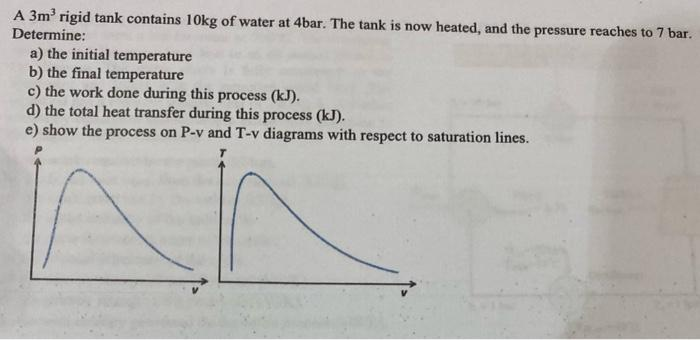Home / Expert Answers / Chemical Engineering / a-3-mathrm-m-3-rigid-tank-contains-10-mathrm-kg-of-water-at-4-mathrm-bar-pa731

# (Solved): A $$3 \mathrm{~m}^{3}$$ rigid tank contains $$10 \mathrm{~kg}$$ of water at $$4 \mathrm{bar} \ ...A \( 3 \mathrm{~m}^{3}$$ rigid tank contains $$10 \mathrm{~kg}$$ of water at $$4 \mathrm{bar}$$. The tank is now heated, and the pressure reaches to 7 bar. Determine: a) the initial temperature b) the final temperature c) the work done during this process $$(\mathrm{kJ}$$ ). d) the total heat transfer during this process $$(\mathrm{kJ})$$. e) show the process on P-v and T-v diagrams with respect to saturation lines.

We have an Answer from Expert